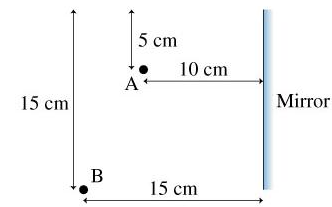# Problem: How far below the top edge does the ray strike the mirror?

###### FREE Expert Solution

Let the distance of the point from the bottom of the mirror to the point be x:

We can calculate x from the triangle properties as:

$\begin{array}{rcl}\frac{\mathbf{10}\mathbf{-}\mathbf{x}}{\mathbf{10}}& \mathbf{=}& \frac{\mathbf{x}}{\mathbf{15}}\\ \mathbf{15}\frac{\mathbf{\left(}\mathbf{10}\mathbf{-}\mathbf{x}\mathbf{\right)}}{\mathbf{10}}& \mathbf{=}& \mathbf{x}\\ \mathbf{150}\mathbf{-}\mathbf{15}\mathbf{x}& \mathbf{=}& \mathbf{10}\mathbf{x}\\ \mathbf{25}\mathbf{x}& \mathbf{=}& \mathbf{150}\end{array}$###### Problem Details

How far below the top edge does the ray strike the mirror?